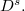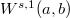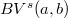﻿

### Bilateral Riemann-Liouville Fractional Sobolev spaces

#### Abstract

We establish some notation and properties of the bilateral Riemann-Liouville fractional derivative$D^s.$ We introduce the associated Sobolev spaces of fractional order$s$, denoted by$W^{s,1}(a,b)$, and the Bounded Variation spaces of fractional order$s$, denoted by$BV^{s}(a,b)$: these spaces are studied with the aim of providing a suitable functional framework for fractional variational models in image analysis.

DOI Code: 10.1285/i15900932v41n2p61

Keywords: Fractional Calculus; Fractional Sobolev and BV Spaces

Full Text: PDF